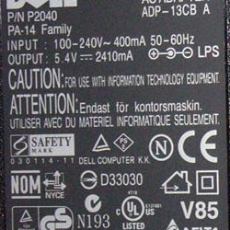## Solar Energy,Power & Electricity

In basic about solar energy, solar power and solar eletricity we will talk about the basic things behind this power. Formulas that will be used to find out which Solar Panel you should use and which battery you should select. And how much Solar Panels do you need to power up lights and other applications. Here are the main things you need to know and that will be used to calculate your needs. AC-DC system, Volt, Current(Ampere), Power(Watt), Resistance, Series and Parallel connecting.#### Parallel Connecting – 12V System

Parallel Connecting solar panels gives higher current. And voltage will remain the same. Parallel Connecting is best for us. Because we do not need high voltage. Normal battery is 12v. And by selecting high voltage require higher voltage charge controller. To connect solar panels in parallel we have to connect plus + to plus and minus – to minus. See the picture for details.

#### Series Connecting – 24V System

By connecting Solar Panels in series connection. It will increase Voltage and current Amps. will remain the same. To connect solar panels in series we have to connect plus + to – minus on next panel. See the picture for details. In this example we have connected 2 solar panels in series which will give 24v output.#### Series and Parallel Connecting – 24v System

If we are creating a 24v system. So then we have to connect two solar panels in series and connect two series connected panels in parallel as showed in figure under. Which increases voltage to 24v and when we have connected two other 24v connected solar panels in parallel so voltage remains same and increases current Amps. If we want to increase output current on 24v so we connect more solar panels in parallel same way under as shown in figure under.

#### AC-DC system

Ac stands for Alternative Current. Alternative current is almost that we found in wall outlet or electric outlet. Clever say’d that we found in wall. It is 230 Volt. DC stands for Direct Current. In solar panels it is used 12 volt dc system. DC is that current we can found in cells, batteries, and using adapters or regulators. See the picture of a dell charger. Dell charger also converts AC Current to DC 5.4 Volt and 2410mA. Solar Panels also uses DC voltage and Current.

#### Volt

Voltage is the electromotive force (pressure) applied to an electrical circuit measured in volts (E).
Example. P=200W, I=4.0A. If we have a value of watt and ampere and we want to find out how much volt does it use then we should use this from Power Circle. E=P/I. 200/4.0=50V. So we found voltage is 50V.

#### Current

Current is the flow of electrons in an electrical circuit measured in amperes (I).
Example. P=100W, E=12V. We want to find out how much ampere does it use. We take a look at circle. I=P/E. 100/12=8.33A. Current usage is 8.33A.

#### Power

Power is the product of the voltage times the current in an electrical circuit measured in watts (P).
Example. E=220V, I=0,4A. This example is taken from picture of Dell Charger. We have 400mA=0,4A. Take a look at circle P=E*I. 220V * 0,4A=88W. Answer is dell charger use 88Watt.#### Resistance

Resistance is the opposition to the flow of electrons in an electrical circuit measured in ohms (R). Increased resistance gives higher voltage and higher power(watt).

Example. E=12V, I=3.0A. We want to find out resistance. We use formula R=E/I. 12V/3.0A = 4 Ohm.